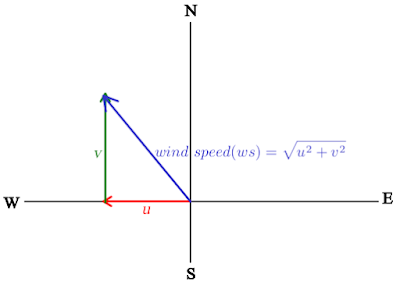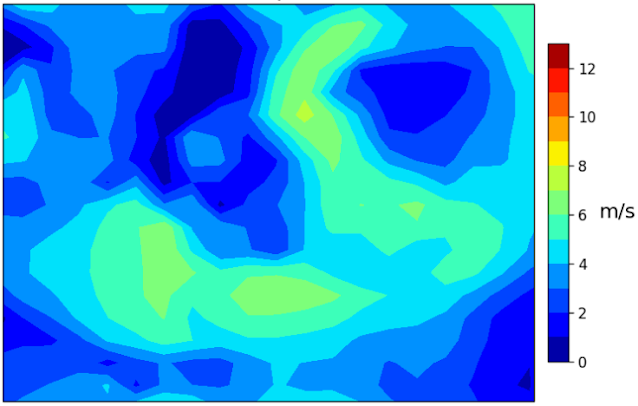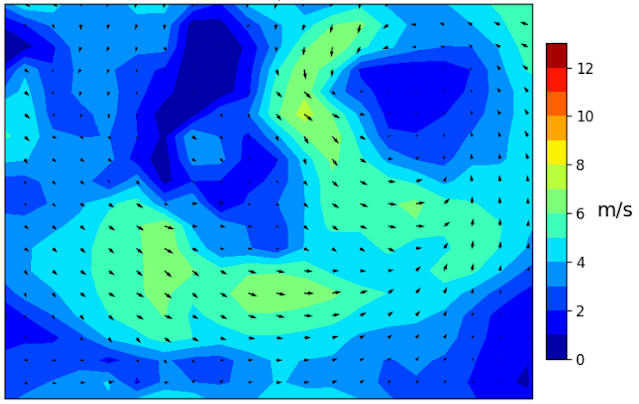# How to Plot Wind Speed and Direction in Python

In the previous post, I discussed creating the time-lapse of Hurricane Harvey's path from radar data, revealing its trajectory as it made landfall in Texas on Friday, August 26, 2017. While the tutorial demonstrated its movement from the early stages until reaching land, it didn't provide information on the wind speed and direction. In this post, we will address that aspect and learn how to plot wind speed and direction in Python. At the end of this tutorial we will get wind speed and direction plot as shown in figure 1.

### Obtaining Wind Data

For this tutorial, we will utilize the second Modern-Era Retrospective analysis for Research and Applications (MERRA-2). MERRA-2, produced by NASA Global Modeling and Assimilation Office (GMAO) using the Goddard Earth Observing System Model (GEOS) version 5.12.4, is the latest version of global atmospheric reanalysis for the satellite era. The dataset covers the period from 1980 to the present, with a latency of approximately three weeks after the end of each month.

To get the data, use this link which will open the MERRA-2 summary page as shown in the figure 2. Before getting the data, you have to do login first and then click Subset/Get Data. If you don't have any account, please create a new one.

After pushing the Subset/Get Data button, a download interface window will appear soon as seen in the figure 3. Here you can define the download method, date range, region, variables to select, time range, etc. The output format is in netCDF.

There are more than 20 variables available from the data. You can get each variable explanation in the summary page. But for our purpose we only need two varibles: 2-meter eastward wind(U2M) and 2-meter northward wind(V2M).

### Plotting Wind Speed and Direction in Python

After getting the data. Let's plot wind speed and direction using Python with the following steps.

Firstly import all libraries that are required such as: netCDF4, numpy, matplotlib, and cartopy. If you don't have a library use pip to install. For example to install the netCDF4 module, in the terminal or command prompt type: pip install netCDF4.

 ```1 2 3 4 5 6 7``` ```#IMPORT LIBRARIES from netCDF4 import Dataset import numpy as np import matplotlib.pyplot as plt import cartopy.crs as ccrs from cartopy.mpl.gridliner import LONGITUDE_FORMATTER, LATITUDE_FORMATTER import matplotlib.ticker as mticker ```

Next, read the NETCDF data using Dataset method from netCDF4 library as in the following code.

 ```1 2 3 4 5``` ```#READ NETCDF DATA path='/home/data/Downloads/' #CHANGE WITH YOURS f_name='MERRA2_400.tavg1_2d_slv_Nx.20230512.SUB.nc' nc_data=path+f_name data = Dataset(nc_data, mode='r') ```

After reading the data, now let's get the component: longitude, latitude, eastward and northward wind speed.

 ``` 1 2 3 4 5 6 7 8 9 10 11``` ```#GETTING THE VARIABLES # longitude and latitude lons = data.variables['lon'] lats = data.variables['lat'] lon, lat = np.meshgrid(lons, lats) # 2-meter eastward wind m/s U2M = data.variables['U2M'] # 2-meter northward wind m/s V2M = data.variables['V2M'] ```

The eastward and northward components are an orthogonal vector. If variables "u" and "v" represent the eastward and northward wind vectors, respectively. The vector u wind aligns with the x-axis (longitude), where positive values indicate westward winds and negative values indicate eastward winds. On the other hand, the v wind aligns with the y-axis (latitude), with positive values indicating winds from the south and negative values indicating winds from the north. By having the u and v wind components, we can calculate the wind speed using the Pythagorean Theorem as illustrate in the figure 4.Figure 4. Wind speed vectors

Simply the wind speed can be calculated using the following code.

 ```1 2``` ```#CALCULATE WIND SPEED ws = np.sqrt(U2M_nans**2+V2M_nans**2) ```

By having the wind speed, let's plot it. To do the plotting we are using the cartopy and matplotlib library with the contourf method as seen at line 10 in the following code. After running the code, we should get the wind speed plot as in figure 5.

 ``` 1 2 3 4 5 6 7 8 9 10 11 12 13 14``` ```#PLOTTING WIND SPEED AND DIRECTION # Set the figure size, projection, and extent fig = plt.figure(figsize=(9,5)) ax = plt.axes(projection=ccrs.PlateCarree()) ax.set_extent([-111.076,-99.283,32.646,41.468]) #SET MAP EXTENT ax.coastlines(resolution="50m",linewidth=1) # Plot windspeed c_inv = np.arange(0,14,1) #contour interval/level plt.contourf(lon, lat, ws[0,:,:], c_inv, transform=ccrs.PlateCarree(),cmap=plt.cm.jet) plt.title('MERRA-2 2m Wind Speed and Direction', size=16) cb = plt.colorbar(ax=ax, orientation="vertical", pad=0.02, aspect=16, shrink=0.8) cb.set_label('m/s',size=14,rotation=0,labelpad=15) cb.ax.tick_params(labelsize=10) ```Figure 5. Wind speed plot

We already get the plot of wind speed that visualize in a contour plot with a color bar legend on the right. Next let's plot the wind direction. To plot the wind direction we use the quiver method as in the following code. In the code below we also save the plot into an image file using savefig method. Running the code the wind direction should be overlaid as shown in the figure 6.

 ```1 2 3 4 5 6``` ```# Overlay wind direction qv = plt.quiver(lon, lat, U2M_nans[0,:,:], V2M_nans[0,:,:], scale=350, color='k') #save plot as image out_path='/media/data/' #Change with yours fig.savefig(out_path+'wind speed and direction.png', format='png', dpi=120) ```Figure 6. Wind speed and direction plot

### Create Wind Speed and Direction Animation

Take a look at line 2 in the code above or this one:

qv = plt.quiver(lon, lat, U2M_nans[0,:,:], V2M_nans[0,:,:], scale=350, color='k')

The variables U2M_nans and V2M_nans have subset [0,:,:], where 0 corresponds to the first layer of the netCDF data. The netCDF dataset comprises a total of 24 layers, numbered from 0 to 23, representing hourly time stamps starting from 00:30 UTC. To obtain data from 1, 2, 3 hours after 00:30 UTC, we should use index 1, 2, 3, and so forth. The same indexing approach applies to the wind speed (ws) variable, which is calculated beforehand.

Using the index in a loop then we can get hourly wind speed and direction. The code below will do it. It will take index from 0 to 23 and save each image.

 ``` 1 2 3 4 5 6 7 8 9 10 11 12 13 14 15 16 17 18 19 20 21 22 23``` ```#PLOTTING WIND SPEED AND DIRECTION IN A LOOP # Set the figure size, projection, and extent fig = plt.figure(figsize=(9,5)) ax = plt.axes(projection=ccrs.PlateCarree()) ax.set_extent([-111.076,-99.283,32.646,41.468]) #SET MAP EXTENT ax.coastlines(resolution="50m",linewidth=1) # Plot windspeed for i in range(24): c_inv = np.arange(0,14,1) #contour interval plt.contourf(lon, lat, ws[i,:,:], c_inv, transform=ccrs.PlateCarree(),cmap=plt.cm.jet) plt.title('Wind Speed and Direction {} hour(s) from 00:30 UTC'.format(i), size=16) if i==0: cb = plt.colorbar(ax=ax, orientation="vertical", pad=0.02, aspect=16, shrink=0.8) cb.set_label('m/s',size=14,rotation=0,labelpad=15) cb.ax.tick_params(labelsize=10) # Overlay wind direction qv = plt.quiver(lon, lat, U2M_nans[i,:,:], V2M_nans[i,:,:], scale=350, color='k') #save plot as image out_path='/media/data/' #Change with yours fig.savefig(out_path+str(i)+'.png', format='png', dpi=120) ```

At the end we can combine all images into an animation that visualize hourly change of wind speed and direction as below. Please take a look this tutorial on how to create map animation.

That's all this tutorial on how to plot wind speed and direction in Python. We had learnt how to obtain wind data from MERRA-2 dataset, read and process the data to calculate wind speed, plotting wind speed and direction and animate the change of wind speed and direction hourly. Hope this tutorial is useful and thank you for reading.

Anyway I provide the code in Jupyter Notebook including the sample data based on request. Make your request here if you are interested.